Java程序设计语言

java 是一种可以撰写跨平台应用软件的面向对象的程序设计语言，是由Sun Microsystems公司于1995年5月推出的Java程序设计语言和Java平台（即JavaEE(j2ee), JavaME(j2me), JavaSE(j2se)）的总称。

1、单链表的创建和遍历

2、求单链表中节点的个数

3、查找单链表中的倒数第k个结点（剑指offer，题15）

4、查找单链表中的中间结点

5、合并两个有序的单链表，合并之后的链表依然有序【出现频率高】（剑指offer，题17）

6、单链表的反转【出现频率最高】（剑指offer，题16）

7、从尾到头打印单链表（剑指offer，题5）

8、判断单链表是否有环

9、取出有环链表中，环的长度

10、单链表中，取出环的起始点（剑指offer，题56）。本题需利用上面的第8题和第9题。

11、判断两个单链表相交的第一个交点（剑指offer，题37）

1、单链表的创建和遍历：

public class LinkList {
public Node current;

//方法：向链表中添加数据
public void add(int data) {
//判断链表为空的时候
if (head == null) {//如果头结点为空，说明这个链表还没有创建，那就把新的结点赋给头结点
head = new Node(data);
} else {
//创建新的结点，放在当前节点的后面（把新的结点合链表进行关联）
current.next = new Node(data);
//把链表的当前索引向后移动一位
current = current.next; //此步操作完成之后，current结点指向新添加的那个结点
}
}

//方法：遍历链表（打印输出链表。方法的参数表示从节点node开始进行遍历
public void print(Node node) {
if (node == null) {
return;
}

current = node;
while (current != null) {
System.out.println(current.data);
current = current.next;
}
}

class Node {
//注：此处的两个成员变量权限不能为private，因为private的权限是仅对本类访问。
int data; //数据域
Node next;//指针域

public Node(int data) {
this.data = data;
}
}

public static void main(String[] args) {
for (int i = 0; i < 10; i++) {
}

}

}

2、求单链表中节点的个数：

//方法：获取单链表的长度
public int getLength(Node head) {
if (head == null) {
return 0;
}

int length = 0;
Node current = head;
while (current != null) {
length++;
current = current.next;
}

return length;
}

3、查找单链表中的倒数第k个结点：

3.1  普通思路：

）。时间复杂度为O（n），大概思路如下：

public int findLastNode(int index) { //index代表的是倒数第index的那个结点

//第一次遍历，得到链表的长度size
if (head == null) {
return -1;
}

while (current != null) {
size++;
current = current.next;
}

//第二次遍历，输出倒数第index个结点的数据
for (int i = 0; i < size - index; i++) {
current = current.next;
}

return current.data;
}

3.2  改进思路：（这种思路在其他题目中也有应用）

这里需要声明两个指针：即两个结点型的变量first和second，首先让first和second都指向第一个结点，然后让second结点往后挪k-1个位置，此时first和second就间隔了k-1个位置，然后整体向后移动这两个节点，直到second节点走到最后一个结点的时候，此时first节点所指向的位置就是倒数第k个节点的位置。时间复杂度为O（n）

public Node findLastNode(Node head, int index) {

if (node == null) {
return null;
}

Node first = head;
Node second = head;

//让second结点往后挪index个位置
for (int i = 0; i < index; i++) {
second = second.next;
}

//让first和second结点整体向后移动，直到second结点为Null
while (second != null) {
first = first.next;
second = second.next;
}

//当second结点为空的时候，此时first指向的结点就是我们要找的结点
return first;
}

要注意k等于0的情况；

如果k大于链表中节点个数时，就会报空指针异常，所以这里需要做一下判断。

public Node findLastNode(Node head, int k) {
if (k == 0 || head == null) {
return null;
}

Node first = head;
Node second = head;

//让second结点往后挪k-1个位置
for (int i = 0; i < k - 1; i++) {
System.out.println("i的值是" + i);
second = second.next;
if (second == null) { //说明k的值已经大于链表的长度了
//throw new NullPointerException("链表的长度小于" + k); //我们自己抛出异常，给用户以提示
return null;
}
}

//让first和second结点整体向后移动，直到second走到最后一个结点
while (second.next != null) {
first = first.next;
second = second.next;
}
//当second结点走到最后一个节点的时候，此时first指向的结点就是我们要找的结点
return first;
}

4、查找单链表中的中间结点：

和上面的第2节一样，也是设置两个指针first和second，只不过这里是，两个指针同时向前走，second指针每次走两步，first指针每次走一步，直到second指针走到最后一个结点时，此时first指针所指的结点就是中间结点。注意链表为空，链表结点个数为1和2的情况。时间复杂度为O（n）。

//方法：查找链表的中间结点
public Node findMidNode(Node head) {

if (head == null) {
return null;
}

Node first = head;
Node second = head;
//每次移动时，让second结点移动两位，first结点移动一位
while (second != null && second.next != null) {
first = first.next;
second = second.next.next;
}

//直到second结点移动到null时，此时first指针指向的位置就是中间结点的位置
return first;
}

5、合并两个有序的单链表，合并之后的链表依然有序：

这道题经常被各公司考察。

1->2->3->4

2->3->4->5

1->2->2->3->3->4->4->5

挨着比较链表1和链表2。

这个类似于归并排序。尤其要注意两个链表都为空、和其中一个为空的情况。只需要O (1) 的空间。时间复杂度为O (max(len1,len2))

//两个参数代表的是两个链表的头结点

if (head1 == null && head2 == null) { //如果两个链表都为空
return null;
}
if (head1 == null) {
}
if (head2 == null) {
}

Node current; //current结点指向新链表
} else {
}

while (head1 != null && head2 != null) {
current.next = head1; //新链表中，current指针的下一个结点对应较小的那个数据
current = current.next; //current指针下移
} else {
current = current.next;
}
}

//合并剩余的元素
if (head1 != null) { //说明链表2遍历完了，是空的
}

if (head2 != null) { //说明链表1遍历完了，是空的
}

}

public static void main(String[] args) {
for (int i = 0; i < 4; i++) {
}

for (int i = 3; i < 8; i++) {
}

}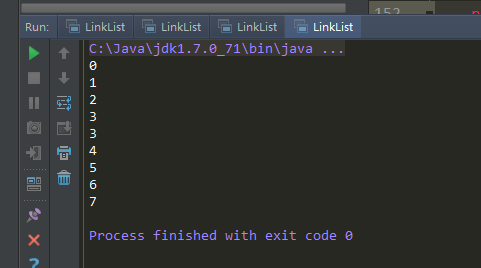6、单链表的反转：【出现频率最高】

1->2->3->4

4->2->2->1

从头到尾遍历原链表，每遍历一个结点，将其摘下放在新链表的最前端。注意链表为空和只有一个结点的情况。时间复杂度为O（n）

//方法：链表的反转
public Node reverseList(Node head) {

//如果链表为空或者只有一个节点，无需反转，直接返回原链表的头结点
if (head == null || head.next == null) {
}

Node current = head;
Node next = null; //定义当前结点的下一个结点
Node reverseHead = null; //反转后新链表的表头

while (current != null) {
next = current.next; //暂时保存住当前结点的下一个结点，因为下一次要用

current.next = reverseHead; //将current的下一个结点指向新链表的头结点

current = next; // 操作结束后，current节点后移
}

}

7、从尾到头打印单链表：

对于这种颠倒顺序的问题，我们应该就会想到栈，后进先出。所以，这一题要么自己使用栈，要么让系统使用栈，也就是递归。注意链表为空的情况。时间复杂度为O（n）

注：不要想着先将单链表反转，然后遍历输出，这样会破坏链表的结构，不建议。

//方法：从尾到头打印单链表
public void reversePrint(Node head) {

if (head == null) {
return;
}

Stack<Node> stack = new Stack<Node>(); //新建一个栈
Node current = head;

//将链表的所有结点压栈
while (current != null) {-
stack.push(current); //将当前结点压栈
current = current.next;
}

//将栈中的结点打印输出即可
while (stack.size() > 0) {
System.out.println(stack.pop().data); //出栈操作
}
}

public void reversePrint(Node head) {

if (head == null) {
return;
}
}

8、判断单链表是否有环：

这里也是用到两个指针，如果一个链表有环，那么用一个指针去遍历，是永远走不到头的。

因此，我们用两个指针去遍历：first指针每次走一步，second指针每次走两步，如果first指针和second指针相遇，说明有环。时间复杂度为O (n)。

//方法：判断单链表是否有环
public boolean hasCycle(Node head) {

if (head == null) {
return false;
}

Node first = head;
Node second = head;

while (second != null) {
first = first.next; //first指针走一步
second = second.next.next; second指针走两步

if (first == second) { //一旦两个指针相遇，说明链表是有环的
return true;
}
}

return false;
}

public class LinkList {
public Node current;

//方法：向链表中添加数据
public void add(int data) {
//判断链表为空的时候
if (head == null) {//如果头结点为空，说明这个链表还没有创建，那就把新的结点赋给头结点
head = new Node(data);
} else {
//创建新的结点，放在当前节点的后面（把新的结点合链表进行关联）
current.next = new Node(data);
//把链表的当前索引向后移动一位
current = current.next;
}
}

//方法重载：向链表中添加结点
public void add(Node node) {
if (node == null) {
return;
}

if (head == null) {
} else {
current.next = node;
current = current.next;
}
}

//方法：遍历链表（打印输出链表。方法的参数表示从节点node开始进行遍历
public void print(Node node) {
if (node == null) {
return;
}

current = node;
while (current != null) {
System.out.println(current.data);
current = current.next;
}
}

//方法：检测单链表是否有环
public boolean hasCycle(Node head) {

if (head == null) {
return false;
}

Node first = head;
Node second = head;

while (second != null) {
first = first.next; //first指针走一步
second = second.next.next; //second指针走两步

if (first == second) { //一旦两个指针相遇，说明链表是有环的
return true;
}
}

return false;
}

class Node {
//注：此处的两个成员变量权限不能为private，因为private的权限是仅对本类访问。
int data; //数据域
Node next;//指针域

public Node(int data) {
this.data = data;
}
}

public static void main(String[] args) {
for (int i = 0; i < 4; i++) {
}

}
}

88行：我们将头结点继续往链表中添加，此时单链表就环了。最终运行效果为true。

9、取出有环链表中，环的长度：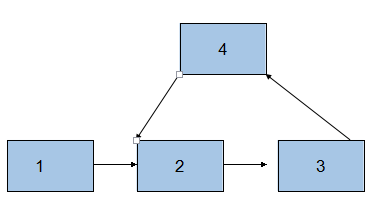这里面，我们需要先利用上面的第7小节中的hasCycle方法（判断链表是否有环的那个方法），这个方法的返回值是boolean型，但是现在要把这个方法稍做修改，让其返回值为相遇的那个结点。然后，我们拿到这个相遇的结点就好办了，这个结点肯定是在环里嘛，我们可以让这个结点对应的指针一直往下走，直到它回到原点，就可以算出环的长度了。

//方法：判断单链表是否有环。返回的结点是相遇的那个结点
public Node hasCycle(Node head) {

if (head == null) {
return null;
}

Node first = head;
Node second = head;

while (second != null) {
first = first.next;
second = second.next.next;

if (first == second) { //一旦两个指针相遇，说明链表是有环的
return first; //将相遇的那个结点进行返回
}
}
return null;
}

//方法：有环链表中，获取环的长度。参数node代表的是相遇的那个结点
public int getCycleLength(Node node) {

if (head == null) {
return 0;
}

Node current = node;
int length = 0;

while (current != null) {
current = current.next;
length++;
if (current == node) { //当current结点走到原点的时候
return length;
}
}
return length;
}

public class LinkList {
public Node current;

public int size;

//方法：向链表中添加数据
public void add(int data) {
//判断链表为空的时候
if (head == null) {//如果头结点为空，说明这个链表还没有创建，那就把新的结点赋给头结点
head = new Node(data);
} else {
//创建新的结点，放在当前节点的后面（把新的结点合链表进行关联）
current.next = new Node(data);
//把链表的当前索引向后移动一位
current = current.next; //此步操作完成之后，current结点指向新添加的那个结点
}
}

//方法重载：向链表中添加结点
public void add(Node node) {
if (node == null) {
return;
}
if (head == null) {
} else {
current.next = node;
current = current.next;
}
}

//方法：遍历链表（打印输出链表。方法的参数表示从节点node开始进行遍历
public void print(Node node) {
if (node == null) {
return;
}

current = node;
while (current != null) {
System.out.println(current.data);
current = current.next;
}
}

//方法：判断单链表是否有环。返回的结点是相遇的那个结点
public Node hasCycle(Node head) {

if (head == null) {
return null;
}

Node first = head;
Node second = head;

while (second != null) {
first = first.next;
second = second.next.next;

if (first == second) { //一旦两个指针相遇，说明链表是有环的
return first; //将相遇的那个结点进行返回
}
}

return null;
}

//方法：有环链表中，获取环的长度。参数node代表的是相遇的那个结点
public int getCycleLength(Node node) {

if (head == null) {
return 0;
}

Node current = node;
int length = 0;

while (current != null) {
current = current.next;
length++;
if (current == node) { //当current结点走到原点的时候
return length;
}
}

return length;
}

class Node {
//注：此处的两个成员变量权限不能为private，因为private的权限是仅对本类访问。
int data; //数据域
Node next;//指针域

public Node(int data) {
this.data = data;
}
}

public static void main(String[] args) {

Node second = null; //把第二个结点记下来

for (int i = 0; i < 4; i++) {
if (i == 1) {
second = list1.current; //把第二个结点记下来
}
}

Node current = list1.hasCycle(list1.head); //获取相遇的那个结点

System.out.println("环的长度为" + list1.getCycleLength(current));
}

}

运行效果：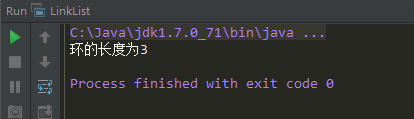public static void main(String[] args) {
for (int i = 0; i < 4; i++) {
}

Node current = list1.hasCycle(list1.head);

System.out.println("环的长度为" + list1.getCycleLength(current));
}10、单链表中，取出环的起始点：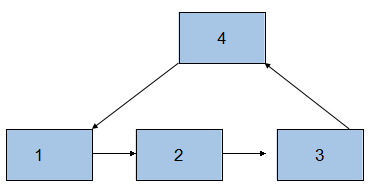//方法：获取环的起始点。参数length表示环的长度
public Node getCycleStart(Node head, int cycleLength) {

if (head == null) {
return null;
}

Node first = head;
Node second = head;
//先让second指针走length步
for (int i = 0; i < cycleLength; i++) {
second = second.next;
}

//然后让first指针和second指针同时各走一步
while (first != null && second != null) {
first = first.next;
second = second.next;

if (first == second) { //如果两个指针相遇了，说明这个结点就是环的起始点
return first;
}
}

return null;
}

public class LinkList {
public Node current;

public int size;

//方法：向链表中添加数据
public void add(int data) {
//判断链表为空的时候
if (head == null) {//如果头结点为空，说明这个链表还没有创建，那就把新的结点赋给头结点
head = new Node(data);
} else {
//创建新的结点，放在当前节点的后面（把新的结点合链表进行关联）
current.next = new Node(data);
//把链表的当前索引向后移动一位
current = current.next; //此步操作完成之后，current结点指向新添加的那个结点
}
}

//方法重载：向链表中添加结点
public void add(Node node) {
if (node == null) {
return;
}
if (head == null) {
} else {
current.next = node;
current = current.next;
}
}

//方法：遍历链表（打印输出链表。方法的参数表示从节点node开始进行遍历
public void print(Node node) {
if (node == null) {
return;
}

current = node;
while (current != null) {
System.out.println(current.data);
current = current.next;
}
}

//方法：判断单链表是否有环。返回的结点是相遇的那个结点
public Node hasCycle(Node head) {

if (head == null) {
return null;
}

Node first = head;
Node second = head;

while (second != null) {
first = first.next;
second = second.next.next;

if (first == second) { //一旦两个指针相遇，说明链表是有环的
return first; //将相遇的那个结点进行返回
}
}

return null;
}
//方法：有环链表中，获取环的长度。参数node代表的是相遇的那个结点
public int getCycleLength(Node node) {

if (head == null) {
return 0;
}

Node current = node;
int length = 0;

while (current != null) {
current = current.next;
length++;
if (current == node) { //当current结点走到原点的时候
return length;
}
}

return length;
}

//方法：获取环的起始点。参数length表示环的长度
public Node getCycleStart(Node head, int cycleLength) {

if (head == null) {
return null;
}

Node first = head;
Node second = head;
//先让second指针走length步
for (int i = 0; i < cycleLength; i++) {
second = second.next;
}

//然后让first指针和second指针同时各走一步
while (first != null && second != null) {
first = first.next;
second = second.next;

if (first == second) { //如果两个指针相遇了，说明这个结点就是环的起始点
return first;
}
}

return null;
}
class Node {
//注：此处的两个成员变量权限不能为private，因为private的权限是仅对本类访问。
int data; //数据域
Node next;//指针域

public Node(int data) {
this.data = data;
}
}

public static void main(String[] args) {

Node second = null; //把第二个结点记下来

for (int i = 0; i < 4; i++) {

if (i == 1) {
second = list1.current; //把第二个结点记下来
}
}

Node current = list1.hasCycle(list1.head); //获取相遇的那个结点

int length = list1.getCycleLength(current); //获取环的长度

System.out.println("环的起始点是" + list1.getCycleStart(list1.head, length).data);

}

}

11、判断两个单链表相交的第一个交点：

《编程之美》P193，5.3，面试题37就有这道题。

面试时，很多人碰到这道题的第一反应是：在第一个链表上顺序遍历每个结点，每遍历到一个结点的时候，在第二个链表上顺序遍历每个结点。如果在第二个链表上有一个结点和第一个链表上的结点一样，说明两个链表在这个结点上重合。显然该方法的时间复杂度为O(len1 * len2)。

我们可以看出两个有公共结点而部分重合的链表，拓扑形状看起来像一个Y，而不可能是X型。 如下图所示：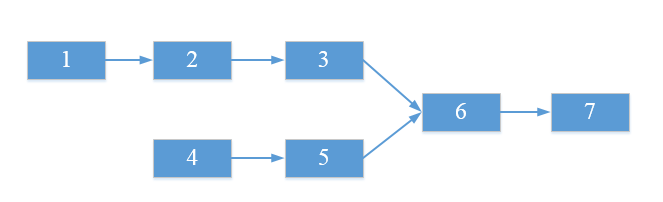//方法：求两个单链表相交的第一个交点
public Node getFirstCommonNode(Node head1, Node head2) {
if (head1 == null || head == null) {
return null;
}

int length1 = getLength(head1);
int length2 = getLength(head2);
int lengthDif = 0; //两个链表长度的差值

//找出较长的那个链表
if (length1 > length2) {
lengthDif = length1 - length2;
} else {
lengthDif = length2 - length1;
}

//将较长的那个链表的指针向前走length个距离
for (int i = 0; i < lengthDif; i++) {
}

//将两个链表的指针同时向前移动
while (longHead != null && shortHead != null) {
}
}

return null;
}

//方法：获取单链表的长度
public int getLength(Node head) {
if (head == null) {
return 0;
}

int length = 0;
Node current = head;   while (current != null) {

length++;
current = current.next;
}

return length;

JAVA实现链表面试题
Java实现栈和队列面试题
Java开发基础笔试题汇总
java开发工程师笔试题汇总

java企业招聘笔试题

10个经典的Java main方法面试题

Java常见面试题二则
JAVA常见面试题总结